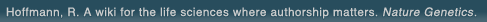# The world's first wiki where authorship really matters (Nature Genetics, 2008). Due credit and reputation for authors. Imagine a global collaborative knowledge base for original thoughts. Search thousands of articles and collaborate with scientists around the globe.

wikigene or wiki gene protein drug chemical gene disease author authorship tracking collaborative publishing evolutionary knowledge reputation system wiki2.0 global collaboration genes proteins drugs chemicals diseases compound
Hoffmann, R. A wiki for the life sciences where authorship matters. Nature Genetics (2008)# Development and validation of computed tomography derived anthropometric regression equations for estimating abdominal adipose tissue distribution.

The purpose of this study was twofold: (1) to develop multiple regression equations for predicting computed tomography (CT) derived intra-abdominal (IAF), subcutaneous ( SCF), and total (TOTF= IAF+SCF) abdominal adipose tissue areas from anthropometric measures in adult white males with a large range of age (18-71 years) and percent body fat (2.0-40.6); and (2) to validate the new and existing equations that used similar Hounsfield Units (HU) for determining IAF for estimating these fat depots. One hundred fifty-one white male subjects had IAF, SCF, and TOTF determined by a single CT scan, skinfold and circumference measures taken and body density determined. Linear intra-correlations and factor analysis procedures were used to identify variables for inclusion in stepwise multiple regression solutions. IAF was estimated from age, waist circumference, the sum of mid-thigh and lower thigh circumferences, and vertical abdominal skinfold. SCF was estimated from age, umbilicus circumference, chest and suprailiac skinfolds. TOTF was estimated from age, body mass index (BMI), chest skinfold, and umbilicus circumference. R2 for IAF, SCF, and TOTF was .73, .77, and .86 respectively. The existing and the new equations were validated on an independent sub-sample of 51 subjects. The only existing equation that met validation criteria had a validation R2 = .67 for IAF. All three new equations met validation criteria with R2 validations of .75, .79, and .85 for IAF, SCF, and TOTF respectively. It is concluded that the new equations might be used as an inexpensive estimation of IAF, SCF, and TOTF in adult white males varying greatly in age and percent body fat.

## References

1. Development and validation of computed tomography derived anthropometric regression equations for estimating abdominal adipose tissue distribution. Kekes-Szabo, T., Hunter, G.R., Nyikos, I., Nicholson, C., Snyder, S., Berland, L. Obes. Res. (1994) [Pubmed]